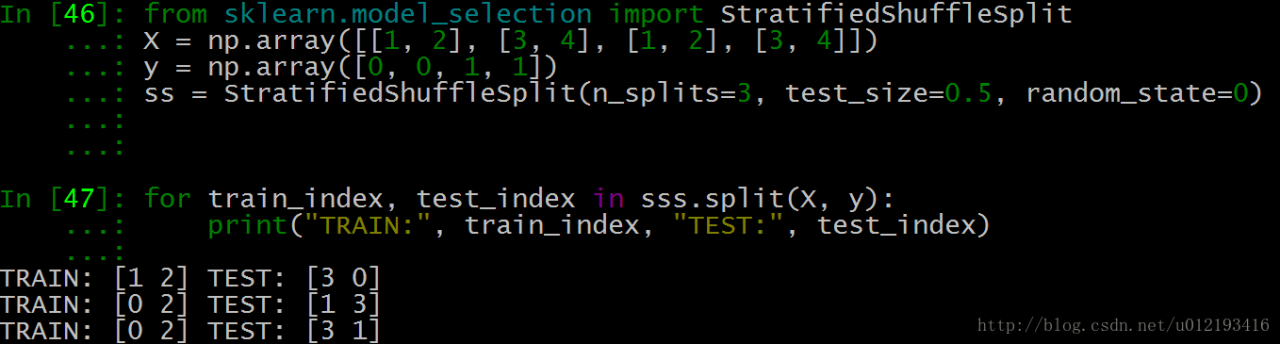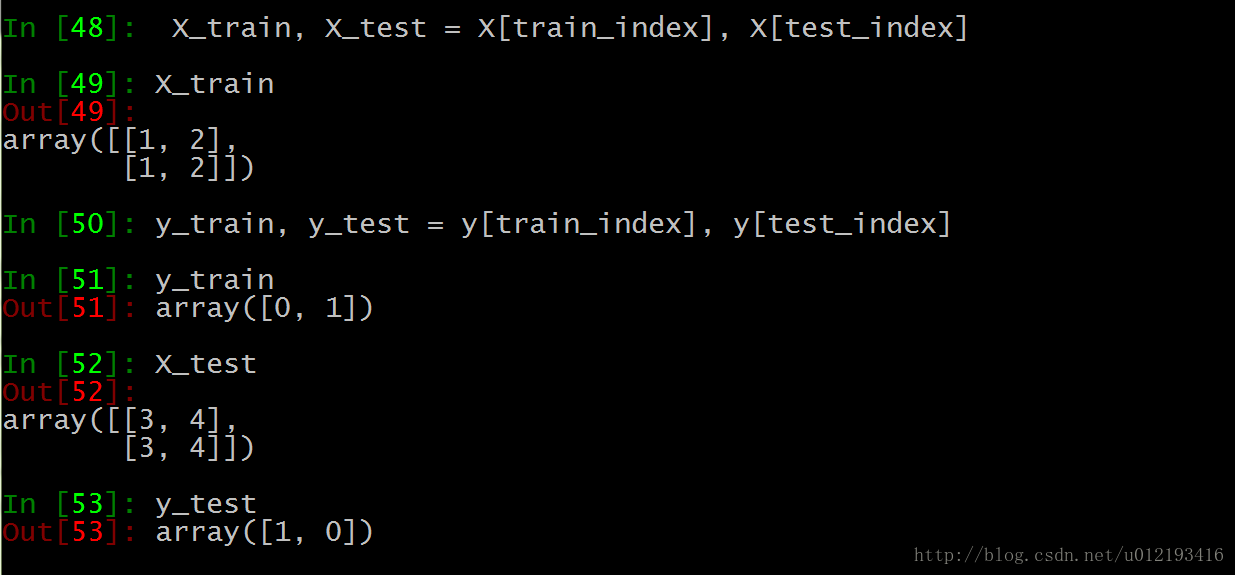# The function of structured shuffleplit() in SK learn realizes the division of data set

Sk learn provides the function of scrambling the data set. Stratifiedsufflesplit() is a very practical function. Before the data set is divided, scrambling operation is the first step. Otherwise, it is easy to produce over fitting and the generalization ability of the model will be reduced.

``sklearn.model_selection.StratifiedShuffleSplit(n_splits=10, test_size=’default’, train_size=None, random_state=None)``

Parameters

n_ splits

It is the number of training data divided into train/test pairs, which can be set according to needs. The default value is 10

Parameter test_ Size and train_ Size is used to set the proportion of train and test in the train/test pair.

Note: Train_ num≥2，test_ num≥2 ;test_ size+train_ Size can be less than 1*

Parameter ﹣ random_ State control is to randomly scramble samples

Function action description
1. It generates a specified number of independent train/test data sets and divides the data sets into n groups.
2. Firstly, the samples are randomly scrambled, and then the train/test pairs are divided according to the set parameters.
3. Each group division created by it will ensure the same analogy proportion of each group. That is to say, if the proportion of the first group of training data categories is 2:1, then each group of the following categories will meet this proportion. Code example:As shown in the above test data, each group of Index Y and index 3 are generated.

test_ Size = 0.5, which means half of the test and training data, and the index values of train and test are 2

n_ Splits = 3, there are three sets of index values

We take the last set of index values:Training set 0 is [1,2], the second is [1,2], and the tag is the corresponding 0,1

The third test set is [3,4], the first is [3,4], and the tag corresponds to 1,0